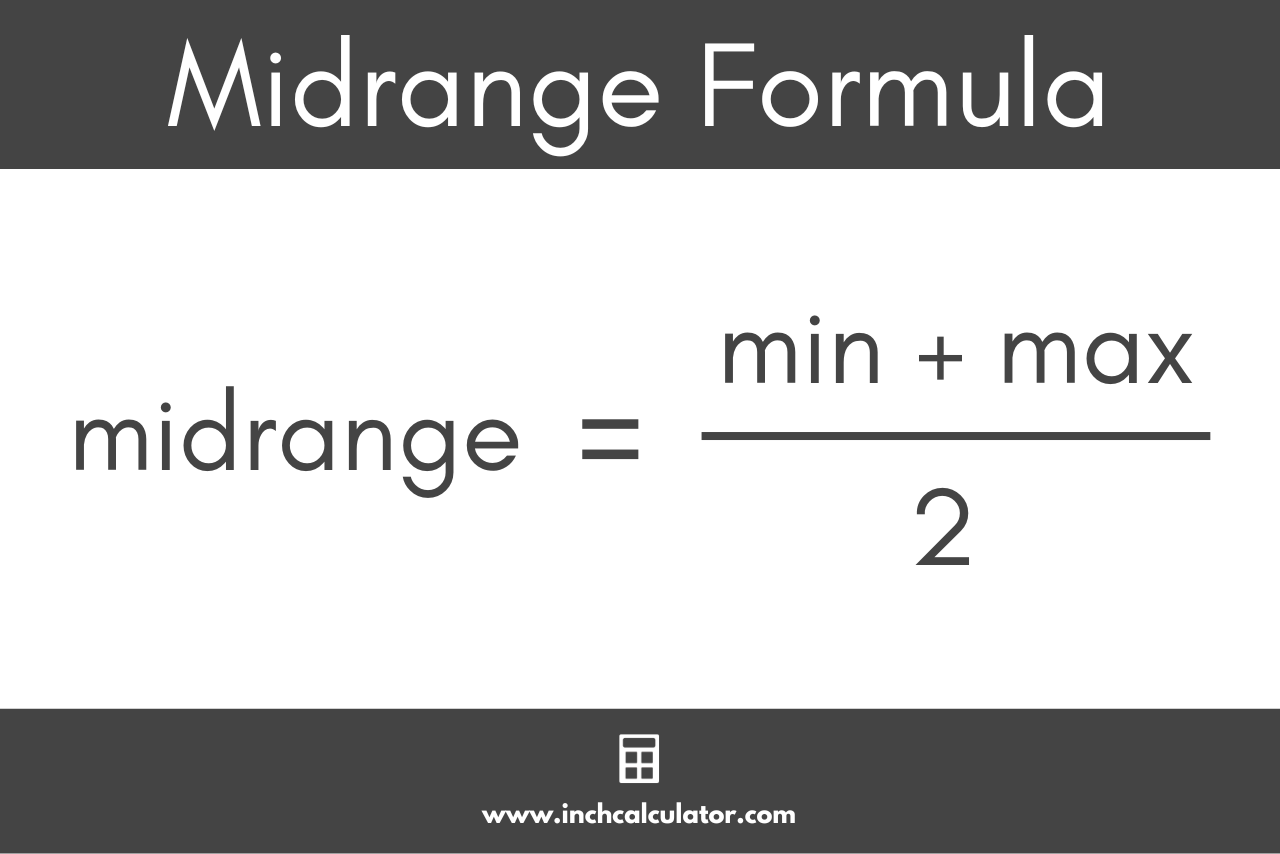# Midrange Calculator

Find the midrange for a set of data by entering the numbers in the calculator below. Keep reading below to learn what the midrange is and how to calculate it.

Separate numbers using a comma (,)

## Results:

### Steps to Solve

#### Step One: Find the Min and Max Values

Start by finding the minimum and maximum values in the dataset.

min = ?

max = ?

#### Step Two: Find the Midrange

Then, apply the midrange formula to solve.

midrange = (min + max) / 2

Learn how we calculated this below

## How to Find the Midrange

In statistics, the midrange, sometimes called the mid-extreme mid-range, is the arithmetic mean of the smallest and largest numbers in a data set. While the range is a measure of the dispersion of values in a data set, the midrange is a measure of center, or central tendency, of the data.

The midrange is not the most accurate indicator of the center of a data set since outliers in the data can significantly change its value. The more commonly used measures of center are the mean, median, and mode.

### Midrange Formula

Since the midrange is the mean of the smallest and largest numbers, the midrange formula is:

midrange = min + max / 2

Thus, the midrange is equal to the highest value (max) in the data plus the lowest value (min), divided by 2.For example: let’s find the midrange for the numbers [13,15,34,41]

lowest number = 13
highest number = 41
midrange = 13 + 41 / 2
midrange = 54 / 2
midrange = 27

The midrange of this data set is 27.

Check out our statistics calculator for more summary statistics measurements.